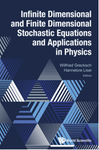• library@msu.ac.th
• Academic Resource Center Mahasarakham University
• library@msu.ac.th
• Academic Resource Center Mahasarakham Universityหมวดหมู่### Infinite dimensional and finite dimensional stochastic equations and applications in physics (E-Book, Multi-user Access)

"This volume contains survey articles on various aspects of stochastic partial differential equations (SPDEs) and their applications in stochastic control theory and in physics. The topics presented in this volume are: -dynamics of stochastic reaction-diffusion equations; -stochastic Itรด-Volterra backward equations in Banach spaces; -stochastic equations of Schrรถdinger type; -optimal control of stochastic Navier-Stokes equations; -quantum Hamilton equations from stochastic optimal control theory. This book is intended not only for graduate students in mathematics or physics, but also for mathematicians, mathematical physicists, theoretical physicists, and science researchers interested in the physical applications of the theory of stochastic processes"--

ใส่ตะกร้า
• ISBN9789811209796
• ประเภทหนังสือ
• ผู้แต่ง Grecksch, Wilfried;Lisei, Hannelore
• สำนักพิมพ์ IG Publishing
• ครั้งที่พิมพ์ 0
• ปีที่พิมพ์2020
• ภาษาภาษาอังกฤษ
• หมวดหมู่วิทยาศาสตร์
###### : ข้อมูลหนังสือ

"This volume contains survey articles on various aspects of stochastic partial differential equations (SPDEs) and their applications in stochastic control theory and in physics. The topics presented in this volume are: -dynamics of stochastic reaction-diffusion equations; -stochastic Itรด-Volterra backward equations in Banach spaces; -stochastic equations of Schrรถdinger type; -optimal control of stochastic Navier-Stokes equations; -quantum Hamilton equations from stochastic optimal control theory. This book is intended not only for graduate students in mathematics or physics, but also for mathematicians, mathematical physicists, theoretical physicists, and science researchers interested in the physical applications of the theory of stochastic processes"--